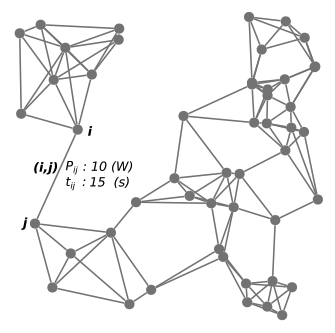# Development of Linear Battery Model for Path Planning with Mixed Integer Linear Programming: Simulated and Experimental Validation### Abstract

Mixed Integer Linear Programs (MILPs) are often used in the path planning of both ground and aerial vehicles. Such a formulation of the path planning problem requires a linear objective function and constraints, limiting the fidelity of the the tracking of vehicle states. One such parameter is the state of charge of the battery used to power the vehicle. Accurate battery state estimation requires nonlinear differential equations to be solved. This state estimation is important in path planning to ensure flyable paths, however when using MILPs to formulate the path planning problem these nonlinear equations cannot be implemented. Poor accuracy in battery estimation during the path planning runs the risk of the planned path being feasible by the estimation model but in reality will deplete the battery to a critical level. To the end of higher accuracy battery estimation within a MILP, we present here a simple linear battery model which predicts the change in state-of-charge (SOC) of a battery given a power draw and duration. This model accounts for changes in battery voltage due to applied electrical load and changes in battery SOC. The battery model is presented and then tested against alternate battery models in numerical and in experimental tests. Further, the effect the proposed linear model has over a simpler SOC estimation on the time-to-solve a resource constrained shortest path problem is also evaluated, implemented in two different algorithms. It is seen that the linear model performs well in battery state estimation while remaining implementable in a Linear Program or MILP, with little affect on the time-to-solve.

Publication
ArXiv